# GSEB Solutions Class 12 Maths Chapter 10 Vector Algebra Miscellaneous Exercise

Gujarat Board GSEB Textbook Solutions Class 12 Maths Chapter 10 Vector Algebra Miscellaneous Exercise Textbook Questions and Answers.

## Gujarat Board Textbook Solutions Class 12 Maths Chapter 10 Vector Algebra Miscellaneous Exercise

Question 1.
Write down a unit vector in XY plane making an angle of 30° with the positive direction of x-axis.
Solution:
Let OX, OY, OZ be the coordinate axes. The line OP lies in the plane XY so that ∠XOP = 30°, ∠POY = 60° and ∠POZ = 90°.
∴ Direction angles of OP are 30°, 60° and 90°.
∴ Direction cosines of OP are cos 30°, cos 60° and cos 90°.Question 2.
Find the scalar components and magnitude of the vector joining the points P(x1, y1, z1) and Q(x2, y2, z2).
Solution:
Positive vectors of P and Q are x1$$\hat {i}$$ + y1$$\hat {j}$$ + z1$$\hat {k}$$
and x2$$\hat {i}$$ + y2$$\hat {j}$$ + z2$$\hat {k}$$ respectively.
∴ $$\overrightarrow{\mathrm{PQ}}$$ = $$\overrightarrow{\mathrm{OQ}}$$ – $$\overrightarrow{\mathrm{OP}}$$
= (x2$$\hat {i}$$ + y2$$\hat {j}$$ + z2$$\hat {k}$$) – (x1$$\hat {i}$$ + y1$$\hat {j}$$ + z1$$\hat {k}$$)
= (x2 – x1)$$\hat {i}$$ + (y2 – y1)$$\hat {j}$$ + (z2 – z1)$$\hat {k}$$
∴ Scalar components of vector $$\overrightarrow{\mathrm{PQ}}$$ are x2 – x1, y2 – y1 and z2 – z1
Further, |$$\overrightarrow{\mathrm{PQ}}$$| = $$\sqrt{\left(x_{2}-x_{1}\right)^{2}+\left(y_{2}-y_{1}\right)^{2}+\left(z_{2}-z_{1}\right)^{2}}$$.

Question 3.
A girl walks 4 km towards west, then she walks 3 km in a direction 30° east of north and stops.
Determine the girl’s displacement from her initial point of departure.
Solution:
The girl walks 4 km westward. This is represented by vector $$\overrightarrow{\mathrm{OQ}}$$ = 4$$\hat {i}$$ …………….. (1)
Further she goes in the direction 30° east of north, i.e., she moves along PQ and stops at Q. PQ makes x-4 an angle of 60° with OP.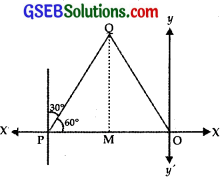Scalar components of PQ along OX = PQ cos 60° = 3 cos 60° = $$\frac{3}{2}$$.
Scalar vertical component of PQ along OY = 3 sin 60° = $$\frac{3 \sqrt{3}}{2}$$.
∴ $$\overrightarrow{\mathrm{PQ}}$$ = $$\frac{3}{2}$$$$\hat {i}$$ + $$\frac{3 \sqrt{3}}{2}$$$$\hat {j}$$ ……………… (2)
Thus, the girl walks along OP and then along PQ.
∴ $$\overrightarrow{\mathrm{OP}}$$ + $$\overrightarrow{\mathrm{PQ}}$$ =$$\overrightarrow{\mathrm{OQ}}$$
From (1) and (2),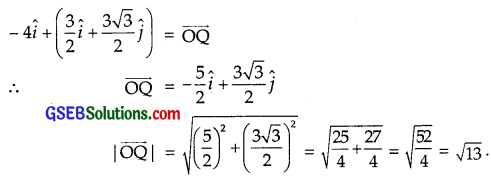Question 4.
If $$\vec {a}$$ = $$\vec {b}$$ + $$\vec {c}$$, then is it true that |$$\vec {a}$$| = |$$\vec {b}$$| + |$$\vec {c}$$|?
Solution:
$$\vec {a}$$ = $$\vec {b}$$ + $$\vec {c}$$
|$$\vec {a}$$| = |$$\vec {b}$$ + $$\vec {c}$$|
∴ |$$\vec {a}$$|2 = |$$\vec {b}$$ + $$\vec {c}$$|2 = ($$\vec {b}$$ + $$\vec {c}$$) . ($$\vec {b}$$ + $$\vec {c}$$)
= $$\vec {b}$$.$$\vec {b}$$ + $$\vec {b}$$.$$\vec {c}$$ + $$\vec {c}$$.$$\vec {b}$$ + $$\vec {c}$$.$$\vec {c}$$
= |$$\vec {b}$$|2 + 2$$\vec {b}$$.$$\vec {c}$$ + |$$\vec {c}$$|2 [∵ $$\vec {b}$$.$$\vec {c}$$ = $$\vec {c}$$.$$\vec {b}$$]
= |$$\vec {b}$$|2 + |$$\vec {c}$$|2 + 2|$$\vec {b}$$| |$$\vec {c}$$| cos θ,
where θ is the angle between $$\vec {b}$$ and $$\vec {c}$$.
When θ = 0° |$$\vec {a}$$|2 = |$$\vec {b}$$|2 + |$$\vec {c}$$|2 + 2|$$\vec {b}$$| |$$\vec {c}$$| = (|$$\vec {b}$$| + |$$\vec {c}$$|2
⇒ |$$\vec {a}$$| = |$$\vec {b}$$| + |$$\vec {c}$$|
If θ ≠ 0°, |$$\vec {a}$$| ≠ |$$\vec {b}$$| + |$$\vec {c}$$|.

Question 5.
Find the value of x for which x ($$\hat {i}$$ + $$\hat {j}$$ + $$\hat {k}$$) is a unit vector.
Solution:
Let $$\vec {a}$$ = x($$\hat {i}$$ + $$\hat {j}$$ + $$\hat {k}$$) = x$$\hat {i}$$ + x$$\hat {j}$$ + x$$\hat {k}$$
|$$\vec {a}$$| = $$\sqrt{x^{2}+x^{2}+x^{2}}$$ = $$\sqrt{3}$$x
But |x| = 1 ⇒ $$\sqrt{3}$$x = ± 1 ∴ x = ± $$\frac{1}{\sqrt{3}}$$.Question 6.
Find a vector of magnitude 5 units and parallel to the resultant of the vectors $$\vec {a}$$ = 2$$\hat {i}$$ + 3$$\hat {j}$$ – $$\hat {k}$$
and $$\vec {b}$$ = $$\hat {i}$$ – 2$$\hat {j}$$ + $$\hat {k}$$.
Solution:
Let vector c be the resultant of $$\vec {a}$$ and $$\vec {b}$$.
Now, $$\vec {c}$$ = $$\vec {a}$$ + $$\vec {b}$$
= (2$$\hat {i}$$ + 3$$\hat {j}$$ – $$\hat {k}$$) + ($$\hat {i}$$ – 2$$\hat {j}$$ + $$\hat {k}$$) = 3$$\hat {i}$$ + $$\hat {j}$$
⇒ |$$\vec {c}$$| = $$\sqrt{3^{2}+1^{2}$$ = $$\sqrt{10}$$.Question 7.
If $$\vec {a}$$ = $$\hat {i}$$ + $$\hat {j}$$ + $$\hat {k}$$, $$\vec {b}$$ = 2$$\hat {i}$$ – $$\hat {j}$$ + 3$$\hat {k}$$
and $$\vec {c}$$ = $$\hat {i}$$ – 2$$\hat {j}$$ + $$\hat {k}$$, find a unit vector parallel to the vector 2$$\vec {a}$$ – $$\vec {b}$$ + 3$$\vec {c}$$.
Solution:
We have:
$$\vec {a}$$ = $$\hat {i}$$ + $$\hat {j}$$ + $$\hat {k}$$, $$\vec {b}$$ = 2$$\hat {i}$$ – $$\hat {j}$$ + 3$$\hat {k}$$
$$\vec {c}$$ = $$\hat {i}$$ – 2$$\hat {j}$$ + $$\hat {k}$$
∴ $$\vec {d}$$ – 2$$\vec {a}$$ – $$\vec {b}$$ + 3$$\vec {c}$$
= 2($$\hat {i}$$ + $$\hat {j}$$ + $$\hat {k}$$) – (2$$\hat {i}$$ – $$\hat {j}$$ + 3$$\hat {k}$$) + 3($$\hat {i}$$ – 2$$\hat {j}$$ + $$\hat {k}$$)
= 2$$\hat {i}$$ + 2$$\hat {j}$$ + 2$$\hat {k}$$ – 2$$\hat {i}$$ + $$\hat {j}$$ – 3$$\hat {k}$$ + 3$$\hat {i}$$ – 6$$\hat {j}$$ + 3$$\hat {k}$$
= (2 – 2 + 3)$$\hat {i}$$ + (2 + 1 – 6)$$\hat {j}$$ + (2 – 3 + 3)$$\hat {k}$$
= 3$$\hat {i}$$ – 3$$\hat {j}$$ + 2$$\hat {k}$$
∴ |$$\vec {d}$$| = $$\sqrt{3^{2}+(-3)^{2}+2^{2}}$$ = $$\sqrt{9+9+4}$$ = $$\sqrt{22}$$.
∴ Unit vector parallel to 2$$\vec {a}$$ – $$\vec {b}$$ + 3$$\vec {c}$$ = $$\frac{3}{\sqrt{22}}$$$$\hat {i}$$ – $$\frac{3}{\sqrt{22}}$$$$\hat {j}$$ + $$\frac{2}{\sqrt{22}}$$$$\hat {k}$$.Question 8.
Show that the points A(1, – 2, – 8), B(5, 0, – 2) and C(11, 3, 7) are collinear and find the ratio in which B divides AC.
Solution:
The position vectors of A and C are $$\hat {i}$$ – 2$$\hat {j}$$ – 8$$\hat {k}$$ and 11$$\hat {i}$$ + 3$$\hat {j}$$ + 7$$\hat {k}$$ respectively.
Let the point B lying on AC divides it in ratio λ : 1
∴ Position vector of B is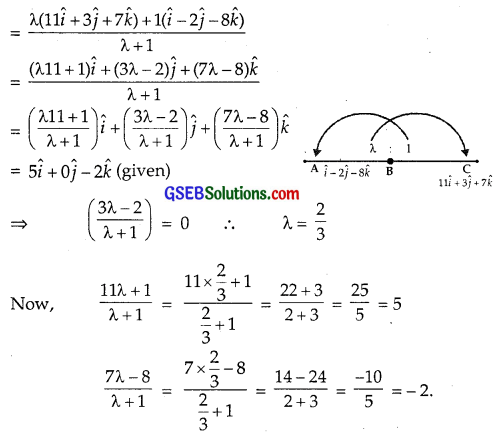⇒ A, B and C are collinear and B divides it in the ratio 2 : 3.

Alternate Method of collinearity:So, A, B and C are collinear.

Question 9.
Find the position vector of a point R which divides the line segment joining the two points P and Q, whose position vectors are (2$$\vec {a}$$ + $$\vec {b}$$) and ($$\vec {a}$$ – 3$$\vec {b}$$),
externally in the ratio 1 : 2. Also, show that P is the middle point of line segment RQ.
Solution:
The position vector of P and Q are respectively (2$$\vec {a}$$ + $$\vec {b}$$) and ($$\vec {a}$$ – 3$$\vec {b}$$).
R divides PQ externally in the ratio 1 : 2.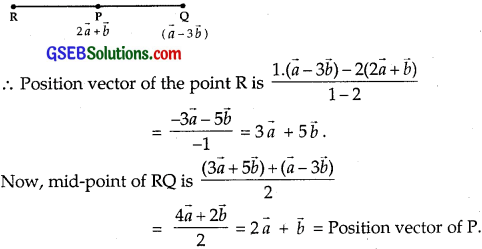⇒ P is the mid-point of RQ and the point R is 3$$\vec {a}$$ + 5$$\vec {b}$$.Question 10.
Two adjacent sides of a parallelogram are 2$$\hat {i}$$ – 4$$\hat {j}$$ + 5$$\hat {k}$$ and $$\hat {i}$$ – 2$$\hat {j}$$ – 3$$\hat {k}$$.
Find the unit vector parallel to its diagonal. Also, find its area.
Solution:
The adjacent sides of a parallelogram are $$\vec {a}$$ = 2$$\hat {i}$$ – 4$$\hat {j}$$ + 5$$\hat {k}$$ and $$\vec {b}$$ = $$\hat {i}$$ – 2$$\hat {j}$$ – 3$$\hat {k}$$.
∴ Its diagonal is $$\vec {a}$$ + $$\vec {b}$$ = (2$$\hat {i}$$ – 4$$\hat {j}$$ + 5$$\hat {k}$$) + ($$\hat {i}$$ – 2$$\hat {j}$$ – 3$$\hat {k}$$)
= 3$$\hat {i}$$ – 6$$\hat {j}$$ + 2$$\hat {k}$$.
|$$\vec {a}$$ + $$\vec {b}$$| = |3$$\hat {i}$$ – 6$$\hat {j}$$ + 2$$\hat {k}$$| = $$\sqrt{3^{2}+(-6)^{2}+2^{2}}$$
= $$\sqrt{9+36+4}$$ = 7.
∴ Unit vector parallel to diagonal of the parallelogram is $$\frac{1}{7}$$(3$$\hat {i}$$ – 6$$\hat {j}$$ + 2$$\hat {k}$$) or $$\frac{3}{7}$$$$\hat {i}$$ – $$\frac{6}{7}$$$$\hat {j}$$ + $$\frac{2}{7}$$$$\hat {k}$$.Thus, the unit vector parallel to its diagonal is $$\frac{1}{7}$$(3$$\hat {i}$$ – 6$$\hat {j}$$ + 2$$\hat {k}$$) and area of || gm is 11$$\sqrt{5}$$ sq.units.

Question 11.
Show that the direction cosines of a vector equally inclined to the axes OX, OY and OZ are $$\frac{1}{\sqrt{3}}$$, $$\frac{1}{\sqrt{3}}$$ and $$\frac{1}{\sqrt{3}}$$ respectively.
Solution:
Let a1, a2, a3 are the direction ratios of the vector.
Now, vector is equally inclined to the axes.
∴ a1 = a2 = a3 = p (say)
⇒ $$\vec {a}$$ = p$$\hat {i}$$ + p$$\hat {j}$$ + p$$\hat {k}$$Hence, $$\frac{1}{\sqrt{3}}$$, $$\frac{1}{\sqrt{3}}$$, $$\frac{1}{\sqrt{3}}$$ are the direction cosines of the line equally inclined to the axes.Question 12.
Let $$\vec {a}$$ = $$\hat {i}$$ + 4$$\hat {j}$$ + 2$$\hat {k}$$, $$\vec {b}$$ = 3$$\hat {i}$$ – 2$$\hat {j}$$ + 7$$\hat {k}$$ and $$\vec {c}$$ = 2$$\hat {i}$$ – $$\hat {j}$$ + 4$$\hat {k}$$.
Find a vector $$\vec {d}$$ which is perpendicular to both $$\vec {a}$$ and $$\vec {b}$$ and $$\vec {c}$$.$$\vec {d}$$ = 15.
Solution:
Let the vector $$\vec {d}$$ be d1$$\hat {i}$$ + d2$$\hat {j}$$ + d3$$\hat {k}$$.
Vector $$\vec {d}$$ is perpendicular to vector $$\vec {a}$$ = $$\hat {i}$$ + 4$$\hat {j}$$ + 2$$\hat {k}$$
⇒ d1 + 4d2 + 2d3 = 0 ……………… (1)
Again vector $$\vec {d}$$ is perpendicular to vector $$\vec {b}$$ = 3$$\hat {i}$$ – 2$$\hat {j}$$ + 7$$\hat {k}$$
∴ 3d1 – 2d2 + 7d3 = 0 ……………….. (2)
From (1) and (2),Question 13.
The scalar product of vector $$\hat {i}$$ + $$\hat {j}$$ + $$\hat {k}$$ with a unit vector along the sum of vectors
2$$\hat {i}$$ + 4$$\hat {j}$$ – 5$$\hat {k}$$ and λ$$\hat {i}$$ + 2$$\hat {j}$$ + 3$$\hat {k}$$ is equal to 1. Find the value of λ.
Solution:
Sum of the vectors 2$$\hat {i}$$ + 4$$\hat {j}$$ – 5$$\hat {k}$$ and λ$$\hat {i}$$ + 2$$\hat {j}$$ + 3$$\hat {k}$$
= (2$$\hat {i}$$ + 4$$\hat {j}$$ – 5$$\hat {k}$$) + (λ$$\hat {i}$$ + 2$$\hat {j}$$ + 3$$\hat {k}$$)
= (2 + λ)$$\hat {i}$$ + 6$$\hat {j}$$ – 2$$\hat {k}$$ = $$\vec {s}$$ (say)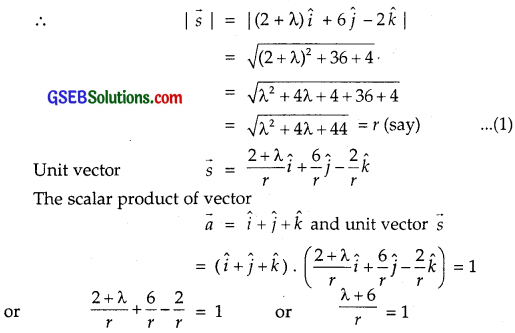⇒ λ + 6 = r
⇒ (λ + 6)2 = r2 = (λ2 + 4λ + 44) [From (1)]
⇒ λ2 + 12λ + 36 = λ2 + 4λ + 44
⇒ 8λ = 44 – 36 = 8
⇒ λ = 1.Question 14.
If $$\vec {a}$$, $$\vec {b}$$ and $$\vec {c}$$ are mutually perpendicular vectors of equal magnitude, show that the vectors $$\vec {a}$$ + $$\vec {b}$$ + $$\vec {c}$$ is equally inclined to $$\vec {a}$$, $$\vec {b}$$ and $$\vec {c}$$.
Solution:
|$$\vec {a}$$| = |$$\vec {b}$$| = |$$\vec {c}$$| = λ(say) ……………… (1)
Also, $$\vec {a}$$, $$\vec {b}$$ and $$\vec {c}$$ are mutually perpendicular.
∴ $$\vec {a}$$.$$\vec {b}$$ = 0, $$\vec {b}$$.$$\vec {c}$$ = 0 and $$\vec {a}$$ + $$\vec {c}$$ = 0 ……………… (2)
Let θ be the angle between vectors $$\vec {a}$$ and $$\vec {a}$$ + $$\vec {b}$$ + $$\vec {c}$$.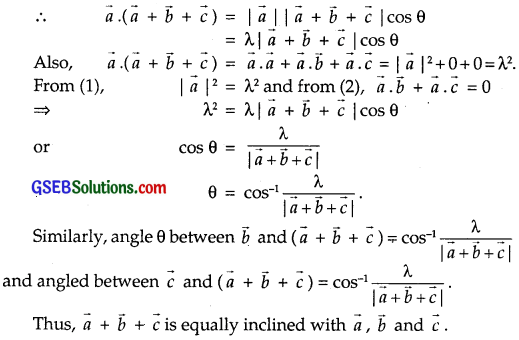Question 15.
Prove that ($$\vec {a}$$ + $$\vec {b}$$).($$\vec {a}$$ + $$\vec {b}$$) = |$$\vec {a}$$|2 + |$$\vec {b}$$|2,
if any and only if $$\vec {a}$$ and $$\vec {b}$$ are perpendicular to each other, given that $$\vec {a}$$ ≠ 0, $$\vec {b}$$ ≠ 0.
Solution:
($$\vec {a}$$ + $$\vec {b}$$).($$\vec {a}$$ + $$\vec {b}$$) = $$\vec {a}$$.($$\vec {a}$$ + $$\vec {b}$$) + $$\vec {b}$$.($$\vec {a}$$ + $$\vec {b}$$)
= $$\vec {a}$$.$$\vec {a}$$ + $$\vec {a}$$.$$\vec {b}$$ + $$\vec {b}$$.$$\vec {a}$$ + $$\vec {b}$$.$$\vec {b}$$
= |$$\vec {a}$$|2 + $$\vec {a}$$.$$\vec {b}$$ + $$\vec {b}$$.$$\vec {a}$$ + |$$\vec {b}$$|2
= |$$\vec {a}$$|2 + |$$\vec {b}$$|2 + 2$$\vec {a}$$$$\vec {b}$$ [∵ $$\vec {b}$$.$$\vec {a}$$ = $$\vec {a}$$.$$\vec {b}$$

(i) Let $$\vec {a}$$ ⊥ $$\vec {b}$$. So, $$\vec {a}$$.$$\vec {b}$$ = 0.
∴ ($$\vec {a}$$ + $$\vec {b}$$).($$\vec {a}$$ + $$\vec {b}$$) = |$$\vec {a}$$|2 + |$$\vec {b}$$|2 + 2$$\vec {a}$$ $$\vec {b}$$
= |$$\vec {a}$$|2 + |$$\vec {b}$$|2 [∵ $$\vec {a}$$.$$\vec {b}$$ = 0]
Again if $$\vec {a}$$ is not perpendicular to $$\vec {b}$$ ⇒ $$\vec {a}$$.$$\vec {b}$$ ≠ 0.
Now, ($$\vec {a}$$ + $$\vec {b}$$).($$\vec {a}$$ + $$\vec {b}$$) = |$$\vec {a}$$|2 + |$$\vec {b}$$|2 + 2 $$\vec {a}$$ $$\vec {b}$$
≠ |$$\vec {a}$$|2 + |$$\vec {b}$$|2
unless $$\vec {a}$$.$$\vec {b}$$ = 0.
Hence, $$\vec {a}$$.$$\vec {b}$$ = 0 or vector $$\vec {a}$$ is perpendicular to $$\vec {b}$$.Choose the correct answers in questions 16 to 19:

Question 16.
If θ is the angle between two vectors $$\vec {a}$$ and $$\vec {b}$$, then $$\vec {a}$$, $$\vec {b}$$ ≥ 0 only, when
(A) 0 < θ < $$\frac{π}{2}$$
(B) θ = $$\frac{π}{3}$$
(C) θ = $$\frac{π}{2}$$
(D) 0 ≤ θ ≤ π
Solution:
$$\vec {a}$$.$$\vec {b}$$ ≥ 0 ⇒ |$$\vec {a}$$| |$$\vec {b}$$| cos θ ≥ 0
Now, |$$\vec {a}$$| = +ve, |$$\vec {b}$$| = +ve ⇒ cos θ ≥ 0
Therefore, 0 ≤ θ ≤ $$\frac{π}{2}$$
∴ Part (B) is the correct answer.

Question 17.
Let $$\vec {a}$$ and $$\vec {b}$$ be two unit vectors and θ be the angle between them. Then, $$\vec {a}$$ + $$\vec {b}$$ is a unit vector, if
(A) θ = $$\frac{π}{4}$$
(B) θ = $$\frac{π}{3}$$
(C) θ = $$\frac{π}{2}$$
(D) θ = $$\frac{2π}{3}$$
Solution:
We have:
|$$\vec {a}$$| = 1, |$$\vec {b}$$| = 1
and |$$\vec {a}$$ + $$\vec {b}$$| = 1, or |$$\vec {a}$$ + $$\vec {b}$$|2 = 1.
i.e. ($$\vec {a}$$ + $$\vec {b}$$). ($$\vec {a}$$ + $$\vec {b}$$) = 1
or |$$\vec {a}$$|2 + |$$\vec {b}$$|2 + 2$$\vec {a}$$$$\vec {b}$$ = 1 [ $$\vec {b}$$.$$\vec {a}$$ = $$\vec {a}$$.$$\vec {b}$$]
= 1 + 1 + 2|$$\vec {a}$$| |$$\vec {b}$$| cos θ = 1
[∵ |$$\vec {a}$$| = 1, |$$\vec {b}$$| = 1],
where θ is the angle between $$\vec {a}$$ and $$\vec {b}$$.
∴ 2 + 2 . 1 . cos θ = 1
or 2 cos θ = 1 ⇒ cos θ = – $$\frac{1}{2}$$
⇒ θ = $$\frac{2π}{3}$$.
∴ Part (D) is the correct answer.Question 18.
The value of $$\hat {i}$$.($$\hat {j}$$ × $$\hat {k}$$) + $$\hat {j}$$.($$\hat {i}$$ × $$\hat {j}$$) is
(A) 0
(B) $$\frac{π}{4}$$
(C) $$\frac{π}{2}$$
(D) π
Solution:
($$\hat {i}$$ + $$\hat {j}$$ + $$\hat {k}$$) are the perpendicular unit vectors, we know that
$$\hat {i}$$ × $$\hat {j}$$ = $$\hat {k}$$, $$\hat {j}$$ × $$\hat {k}$$ = $$\hat {i}$$, $$\hat {k}$$ × $$\hat {i}$$ = $$\hat {j}$$
and $$\hat {i}$$.$$\hat {i}$$ = 1, $$\hat {j}$$.$$\hat {j}$$ = 1 and $$\hat {k}$$.$$\hat {k}$$ = 1
⇒ $$\hat {i}$$.($$\hat {j}$$ × $$\hat {k}$$) + $$\hat {j}$$.($$\hat {k}$$ × $$\hat {i}$$) + $$\hat {k}$$.($$\hat {i}$$ × $$\hat {j}$$)
= $$\hat {i}$$.($$\hat {j}$$ × $$\hat {k}$$) – $$\hat {j}$$.($$\hat {k}$$ × $$\hat {i}$$) + $$\hat {k}$$.($$\hat {i}$$ × $$\hat {k}$$)
[∵ $$\hat {i}$$ × $$\hat {k}$$ = – $$\hat {k}$$ × $$\hat {i}$$]
= $$\hat {i}$$.$$\hat {i}$$ – $$\hat {j}$$.$$\hat {j}$$ + $$\hat {k}$$.$$\hat {k}$$ = 1 – 1 + 1 = 1.
∴ Part (C) is the correct answer.Question 19.
If θ is the angle between any two vectors $$\vec {a}$$ and $$\vec {b}$$, then |$$\vec {a}$$.$$\vec {b}$$| = |$$\vec {a}$$ × $$\vec {b}$$|, when θ is equal to
(A) 0
(B) $$\frac{π}{4}$$
(C) $$\frac{π}{2}$$
(D) π
Solution:
θ is angle between vectors $$\vec {a}$$ and $$\vec {b}$$
∴ |$$\vec {a}$$.$$\vec {b}$$| = |$$\vec {a}$$| |$$\vec {b}$$| |cos θ|
and |$$\vec {a}$$ × $$\vec {b}$$| = |$$\vec {a}$$| |$$\vec {b}$$| |sin θ|
We have: |$$\vec {a}$$.$$\vec {b}$$| = |$$\vec {a}$$ × $$\vec {b}$$|
∴ |$$\vec {a}$$| |$$\vec {b}$$| |cos θ| = |$$\vec {a}$$| |$$\vec {b}$$| |sin θ|
⇒ |cos θ| = |sin θ|
or |tan θ| = 1 or tan θ = 1
⇒ θ = $$\frac{π}{4}$$.
∴ Part (B) is the correct answer.